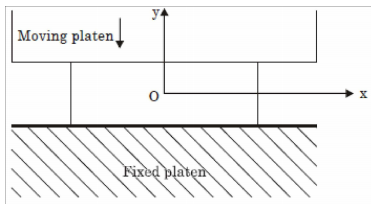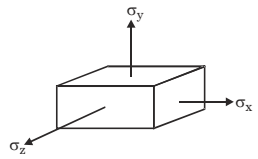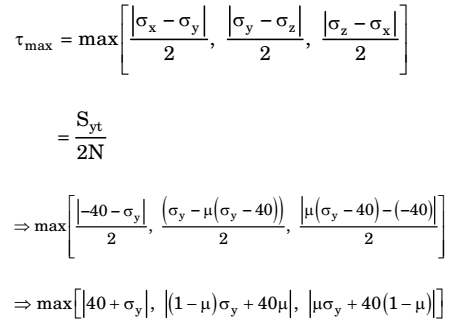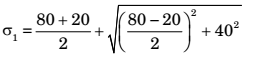## Machine Design Miscellaneous

#### Machine Design

1. A plane-strain compression (forging) of a block is shown in the figure. The strain in the zdirection is zero. The yield strength (Sy) in uniaxial tension/compression of the material of the block is 300 MPa and its follows the Tresca (maximum shear stress) criterion. Assume that the entire block has started yielding. At a point where σx = 40 MPa (compressive) and τxy = 0, the stress component σy is1. σx, σy & σz are principal stresses.Under plane strain condition, εz = 0

 ⇒ σz - μσy - μσx = 0 E E E

⇒ σz = (σx + σy) = μ (σx + σy)
but σx = -40
⇒ σz = μ(-40 + σy)
From Tresca theory,= 300
But μ is not given
Hence if we neglect σz then
max [|40 + σy|, |σy|, |+40|] = 300
⇒ |40 + σy| = 300
40 + σy = 300
⇒ σy = 260 MPa

##### Correct Option: B

σx, σy & σz are principal stresses.Under plane strain condition, εz = 0

 ⇒ σz - μσy - μσx = 0 E E E

⇒ σz = (σx + σy) = μ (σx + σy)
but σx = -40
⇒ σz = μ(-40 + σy)
From Tresca theory,= 300
But μ is not given
Hence if we neglect σz then
max [|40 + σy|, |σy|, |+40|] = 300
⇒ |40 + σy| = 300
40 + σy = 300
⇒ σy = 260 MPa

1. A machine element is subjected to the following bi-axial state of stress; σx = 80 MPa; σy = 20 MPa; τx = 40 MPa. If the shear strength of the material is 100 MPa, the factor of safety as per Tresca's maximum shear stress theory is

1.= 50 + √50² = 100
σ2 = 0

 τ = σ1 - σ2 = 50 7

 FOS = 100 = 2 50

##### Correct Option: B= 50 + √50² = 100
σ2 = 0

 τ = σ1 - σ2 = 50 7

 FOS = 100 = 2 50

1. The uniaxial yield stress of a material is 300 MPa. According to Von Mises criterion, the shear yield stress (in MPa) of the material is _______.

As per Von Mises failure theory
σ²y = σ²1 + σ1σ2 + σ²2
σ1 = – σ2 = τ
then σ²y = τ² + τ² + τ² = 3τ²

 ⇒ τ = σy √3

 Hence τ = σy = 0.5577σy = 173.28 √3

Where τ is shear yield stress

##### Correct Option: A

As per Von Mises failure theory
σ²y = σ²1 + σ1σ2 + σ²2
σ1 = – σ2 = τ
then σ²y = τ² + τ² + τ² = 3τ²

 ⇒ τ = σy √3

 Hence τ = σy = 0.5577σy = 173.28 √3

Where τ is shear yield stress

1. A shaft is subjected to pure torsional moment. The maximum shear stress developed in the shaft is 100 MPa. The yield and ultimate strengths of the shaft material in tension are 300 MPa and 450 MPa, respectively. The factor of safety using maximum distortion energy (Von-Mises) theory is _______ .

1. σ1 = 100 MPa
σ2 = 100 MPa
As per Von-mises yield theory.n² [(σ1 - σ2)² + (σ2 - σ3²) + (σ1 - σ3²)]
≤ 2σ2²
2n²[σ1² + σ2² - σ1σ2] ≤ 2σy²
⇒ n²[(100)² + (100)² + (100)²]≤ (300)²
∴ n = 1.732

##### Correct Option: A

σ1 = 100 MPa
σ2 = 100 MPa
As per Von-mises yield theory.n² [(σ1 - σ2)² + (σ2 - σ3²) + (σ1 - σ3²)]
≤ 2σ2²
2n²[σ1² + σ2² - σ1σ2] ≤ 2σy²
⇒ n²[(100)² + (100)² + (100)²]≤ (300)²
∴ n = 1.732

1. Consider the two states of stress as shown in configurations I and II in the figure below. From the standpoint of distortion energy (vonMises) criterion, which one of the following statements is true?1. Both yields simultaneously.

##### Correct Option: C

Both yields simultaneously.